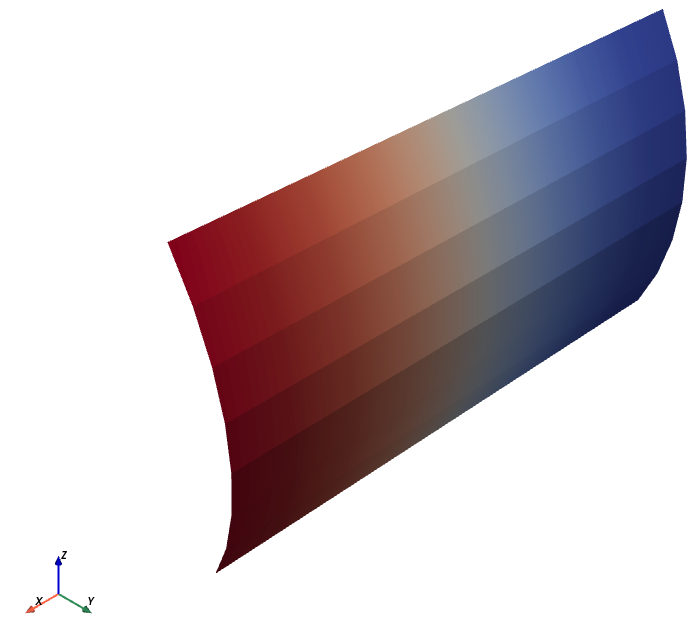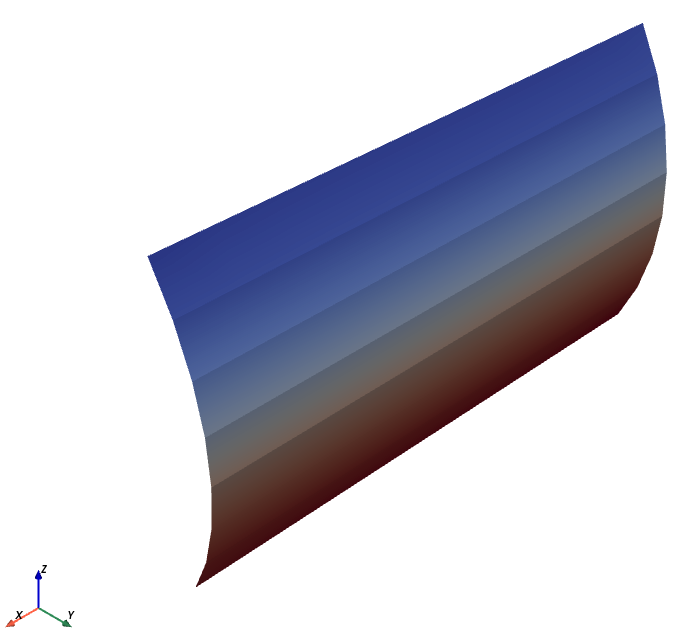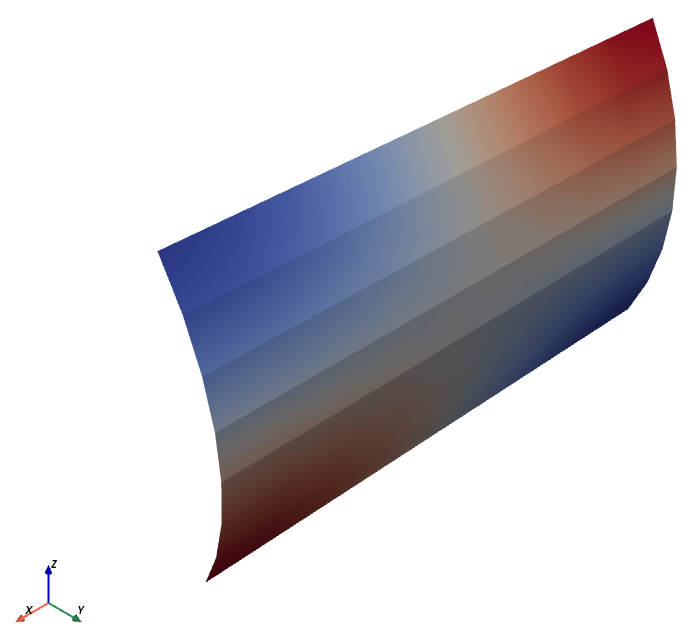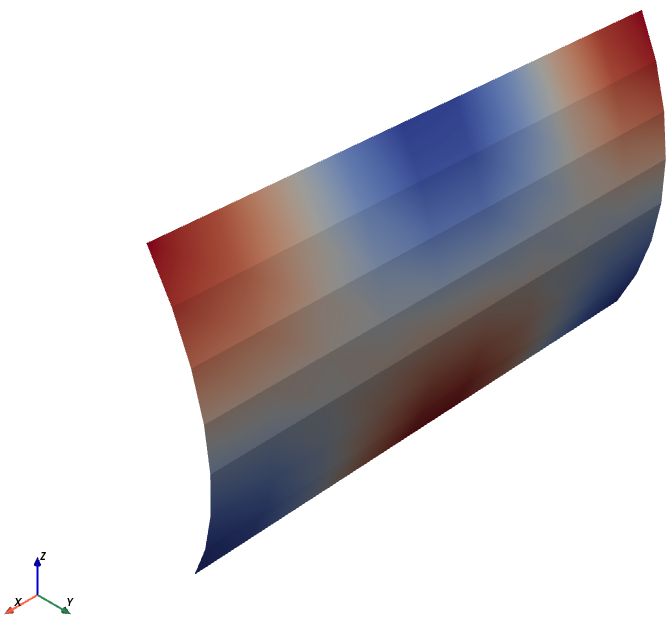# Helmholtz equation in a manifold#

Without any additional effort, we can use our Helmholtz solver in a manifold (see Automating the solution of PDEs on the sphere and other manifolds in FEniCS 1.2).

Let’s start by creating a shell using kokiy:

```from kokiy.axishell import AxiShell

ctrl_pts_x = (0.0, 0.2)
ctrl_pts_r = (0.12, 0.12)
angle = 60
n_azi, n_long = 8, 10

shell = AxiShell(n_azi, n_long, angle, ctrl_pts_x, ctrl_pts_r)
```

Then, we can simply export it directly to a FEniCS readable format:

```shell.export_mesh(mesh_filename, 'triangle')
```

And read it back in FEniCS:

```from dolfin.cpp.mesh import Mesh
from dolfin.cpp.io import XDMFFile

mesh = Mesh()
with XDMFFile(mesh_filename) as file: